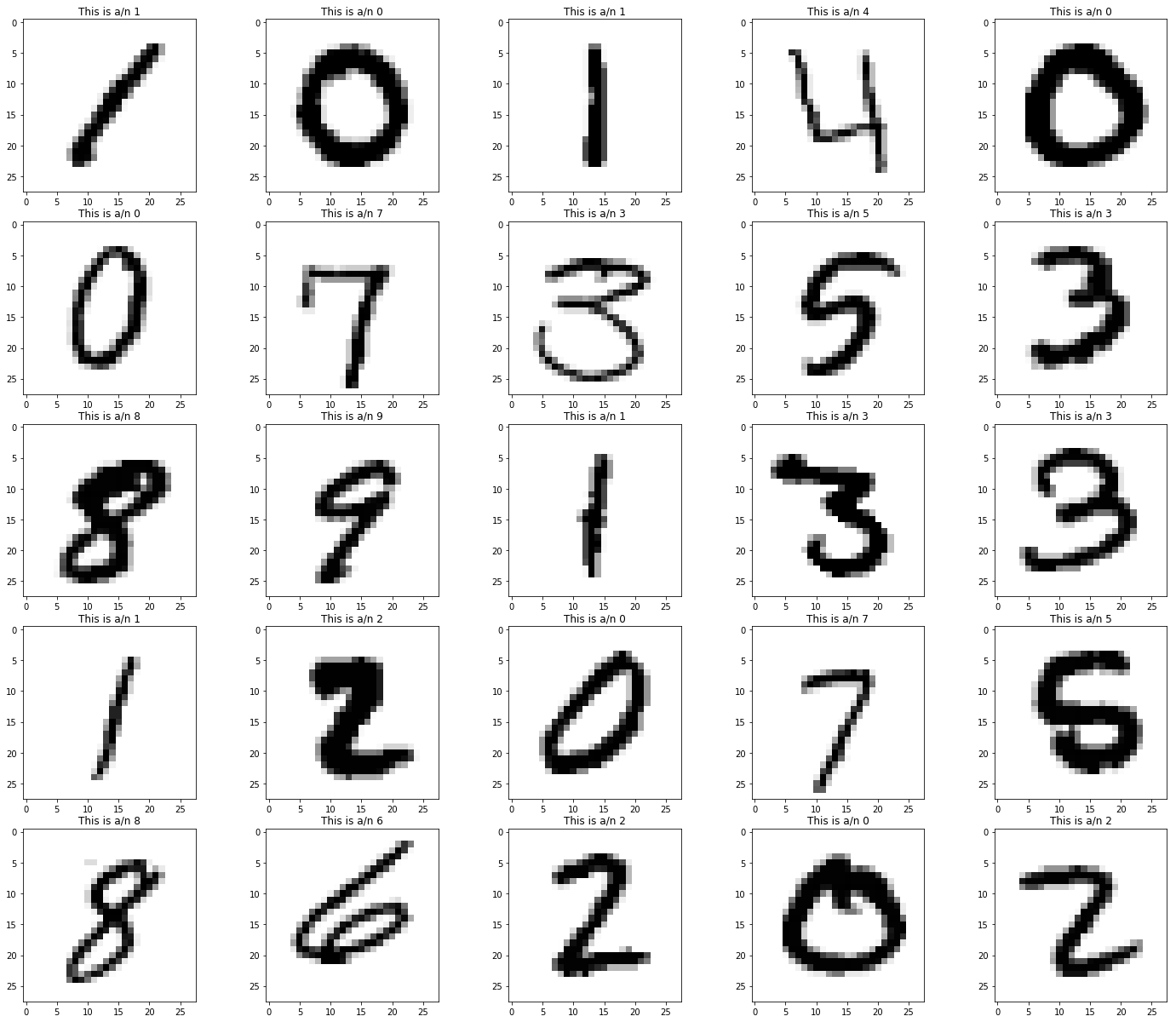## A look at MNIST¶

For those interested in machine learning, the MNIST database of handwritten digits is the most common starting point for classification. The data can be obtained from many places. I downloaded the data files from the kaggle digit-recognizer "competition". This yields a train.csv file and a test.csv file, as well as a sample_submission.csv file that we won't use.

We'll work with train.csv which is a csv file whose rows correspond to labelled images and whose columns are "label" giving the true value of the image and then 784 columns labelled pixelnnn which contain the 0/1 values of a 28x28 image.

In :
import pandas as pd
import matplotlib.pyplot as plt

In :
mnist = pd.read_csv('../data/MNIST/train.csv')
print('We have {} images'.format(mnist.shape))

We have 42000 images


Here are the columns.

In :
mnist.columns

Out:
Index(['label', 'pixel0', 'pixel1', 'pixel2', 'pixel3', 'pixel4', 'pixel5',
'pixel6', 'pixel7', 'pixel8',
...
'pixel774', 'pixel775', 'pixel776', 'pixel777', 'pixel778', 'pixel779',
'pixel780', 'pixel781', 'pixel782', 'pixel783'],
dtype='object', length=785)

This is a little function to draw selections out of the mnist table, just to see how they look.

In :
def show_row(L,ncols=3,size=20):
'''show_row(L,ncols=3,size=20): Display the requested rows (in iterable L) of the mnist dataframe as an image,
using ncols columns, and figsize size.'''
fig, axes = plt.subplots(len(L)//ncols+1,ncols)
fig.set_size_inches(size,size)
N = (len(L)//ncols+1)*ncols
for s,i in enumerate(L):
axes[i//ncols,i%ncols].imshow(mnist.iloc[i,1:].values.reshape(28,28),cmap='gray_r')
axes[i//ncols,i%ncols].set_title('This is a/n {}'.format(mnist.iloc[i,0]))
for j in range(s+1,N):
fig.delaxes(axes[j//ncols,j%ncols])


And here are the first 25 images out of the table.

In :
show_row(range(25),ncols=5)In [ ]: